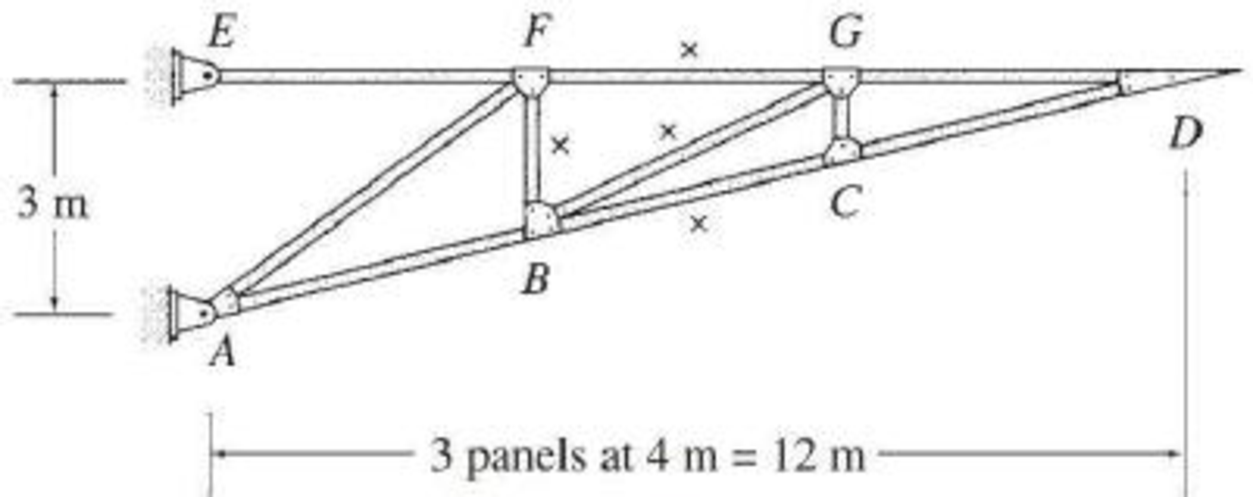# 8.53 through 8.57 Draw the influence lines for the forces in the members identified by an “×” of the trusses shown in Figs. P8.53–P8.57. Live loads are transmitted to the top chords of the trusses. FIG. P8.55

#### Solutions

Chapter
Section
Chapter 8, Problem 55P
Textbook Problem
52 views

## 8.53 through 8.57 Draw the influence lines for the forces in the members identified by an “×” of the trusses shown in Figs. P8.53–P8.57. Live loads are transmitted to the top chords of the trusses.FIG. P8.55To determine

Draw the influence lines for the force in member BC, BF, BG, and FG.

### Explanation of Solution

Calculation:

Find the support reactions.

Apply 1 kN moving load from E to D in the top chord member.

Draw the free body diagram of the member as in Figure 1.

Find the reaction at A and B when 1 kN load placed from E to G. (0x12m).

Apply moment equilibrium at A.

Consider clockwise moment as positive and anticlockwise moment as negative.

ΣMA=0Ex(3)+1(x)=03Ex=xEx=x3

Apply force equilibrium equation along horizontal.

Consider the force acting towards right side as positive (+) and the force acting towards left side as negative ().

ΣFx=0Ax+Ex=0Axx3=0Ax=x3

Consider joint E.

Sketch the free body diagram of the joint E as shown in Figure 2.

Refer Figure 2.

Apply vertical equilibrium at     E.

Consider the upward force as positive (+) and downward force as negative ().

ΣFy=0Ey=0

Refer Figure 1.

Apply vertical equilibrium at     E.

Consider the upward force as positive (+) and downward force as negative ().

ΣFy=0Ay+Ey=1Ay+0=1Ay=1kN

Influence line for the force in member AB.

The expressions for the member force FBC can be determined by passing an imaginary section aa through member FG, BG, and BC and then apply a moment equilibrium at G.

Draw the free body diagram of the section aa as shown in Figure 3.

Refer Figure 3.

Slope of line BC is 4 horizontal and 1 vertical.

Find the equation of member force BG.

Apply a 1 kN load at just left of G (0x8m).

Consider the section GD.

Apply moment equilibrium equation at G.

Consider clockwise moment as positive and anticlockwise moment as negative.

ΣMG=0(442+12)FBC(1)=0FBC=0

Apply a 1 kN load at just right of G (8mx12m).

Consider the section GD

Apply moment equilibrium equation at G.

Consider clockwise moment as positive and anticlockwise moment as negative.

ΣMG=0(442+12)FBC(1)+1(x8)=0417FBC=x+8FBC=174x+217

Thus, the equation of force in the member BC,

FBC=0, 0x8m (1)

FBC=174x+217, 8mx12m (2)

Find the force in member BC using the Equation (1) and (2) and then summarize the value in Table 1.

 x (m) Apply 1 kN load Force in member BC (kN) Influence line ordinate for the force in member BC (kN/kN) 0 E 0 0 4 F 0 0 8 G 0 0 12 D −174(12)+217=4.123 4.123

Sketch the influence line diagram for ordinate for the force in member BC using Table 1 as shown in Figure 4.

Influence line for the force in member FG.

Refer Figure 4.

Find the force in member FG.

Apply 1 kN load just left of F (0x4m).

Consider the section GD.

Apply moment equilibrium equation at B.

Consider clockwise moment as positive and anticlockwise moment as negative.

ΣMB=0FFG(2)=0FFG=0

Apply a 1 kN load at just right of F (4mx12m).

Consider the section FG.

Apply moment equilibrium equation at B.

Consider clockwise moment as positive and anticlockwise moment as negative.

ΣMB=0FFG(2)+1(x4)=02FFG=x4FFG=x22

Thus, the equation of force in the member FG,

FFG=0, 0x4m (3)

FFG=x22, 4mx12m (4)

Find the force in member FG using the Equation (3) and (4) and then summarize the value in Table 2.

 x (m) Apply 1 kN load Force in member FG (kN) Influence line ordinate for the force in member FG (kN/kN) 0 E 0 0 4 F 0 0 8 G 2 2 12 D 122−2=4 4

Sketch the influence line diagram for ordinate for the force in member FG using Table 2 as shown in Figure 5.

Influence line for the force in member BG.

Refer Figure 4.

Slope of member is 4 horizontal and 2 vertical.

Find the force in member BG.

Apply 1 k load just left of F (0x8m)

Consider the section GD.

Apply vertical force equilibrium equation.

Consider the upward force as positive (+) and downward force as negative ()

### Still sussing out bartleby?

Check out a sample textbook solution.

See a sample solution

#### The Solution to Your Study Problems

Bartleby provides explanations to thousands of textbook problems written by our experts, many with advanced degrees!

Get Started

Find more solutions based on key concepts
Which type of lighting system has the highest efficacy?

Engineering Fundamentals: An Introduction to Engineering (MindTap Course List)

Educational requirements for help desk specialists are far more stringent than they are for other careers in th...

Enhanced Discovering Computers 2017 (Shelly Cashman Series) (MindTap Course List)

What is the difference between a threat agent and a threat?

Principles of Information Security (MindTap Course List)

What is a SWOT analysis? Prepare a SWOT analysis of your school or your employer.

Systems Analysis and Design (Shelly Cashman Series) (MindTap Course List)

If your motherboard supports ECC DDR3 memory, can you substitute non-ECC DDR3 memory?

A+ Guide to Hardware (Standalone Book) (MindTap Course List)

What is interpass temperature?

Welding: Principles and Applications (MindTap Course List)